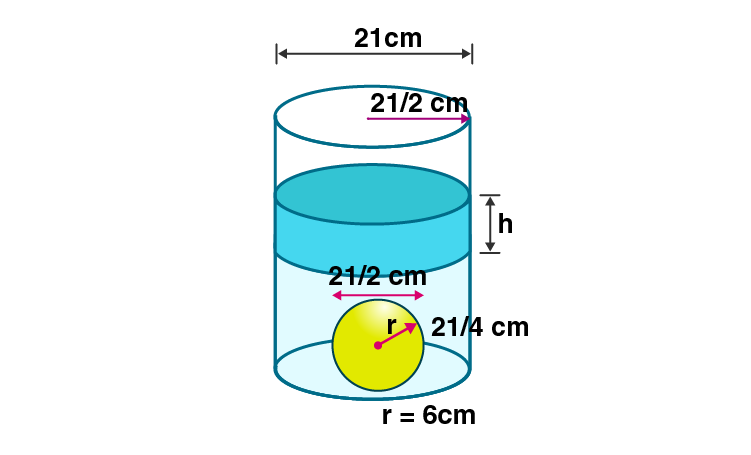Newbie

# A cylindrical can of internal diameter 21 cm contains water. A solid sphere whose diameter is 10.5 cm is lowered into the cylindrical can. The sphere is completely immersed in water. Calculate the rise in water level, assuming that no water overflows.

• 0

This is the Important question of class 10 Based on Mensuration Chapter of M.L Aggarwal book for ICSE BOARD.
A cylindrical can of given internal diameter contains water. A solid sphere whose diameter is given is lowered into the cylindrical can. The sphere is completely immersed in water.
Solve this question in detail.
This is the Question Number 15, Exercise 17.5 of M.L Aggarwal.

Share

1.Given internal diameter of cylindrical can = 21 cm

Radius of the cylindrical can, R = 21/2 cm

Diameter of sphere = 10.5 cm

Radius of the sphere, r = 10.5/2 = 21/4 cm

Let the rise in water level be h.

Rise in volume of water = Volume of sphere immersed

R2h = (4/3)r3

×(21/2)2h = (4/3)××(21/4)3

(21/2) ×(21/2)×h = (4/3)× (21/4)×(21/4)× (21/4)

h = 21/12

h = 7/4

h = 1.75 cm

Hence the rise in water level is 1.75 cm.

• 1